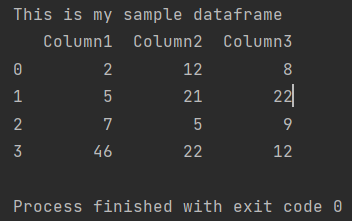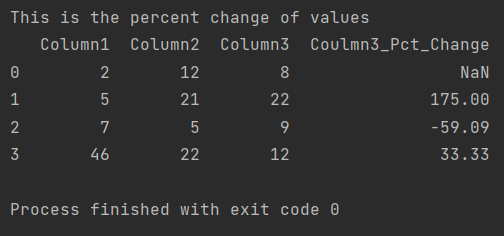Pandas How To Uncategorized How to calculate percent change

# How to calculate percent change

In this post I show you how to calculate percent change in Pandas. You will also learn how to add a percentage change column to the dataframe.

Pandas offers in its wide range of functions one that calculates the percentage change. Use the pct_change function to calculate the percentage change.

## How to calculate percent change in Pandas dataframe

I will use the following dataframe example.

```import pandas as pd

my_df = pd.DataFrame({'Column1': [2, 5, 7, 46],
'Column2': [12, 21, 5, 22],
'Column3': [8, 22, 9, 12]})

print(f'This is my sample dataframe \n{my_df}')

```I would like to calculate the percentage change for column number 3. To calculate the percentage change in column 3, I use the pct_change function.

```import pandas as pd

my_df = pd.DataFrame({'Column1': [2, 5, 7, 46],
'Column2': [12, 21, 5, 22

],
'Column3': [8, 22, 9, 12]})

my_df['Coulmn3_Pct_Change'] = my_df['Column3'].pct_change().round(4)*100
print(f'This is the percent change of values \n{my_df}')

```

The proof that the code is very simple is that I didn’t even use any parameters to the pct_change function.In my Python code, I created a new column in which Pandas calculated the percentage change in the data from column 3. For a neater notation, I multiplied the values by 100 and rounded to two decimal places.

You already know the method to calculate percentage changes in a Pandas dataframe. The pct_change function offers more possibilities. To learn about the possibilities of the pct_change function, I paste the link to the documentation below.

## How to join two dataframes on rowsHow to join two dataframes on rows

You can join two pandas DataFrames along the rows (i.e., concatenate them vertically) using the concat method in pandas. Here’s an example of how to concatenate two DataFrames vertically:

## How to append to empty dataframe in PandasHow to append to empty dataframe in Pandas

To append data to an empty dataframe in Python, you can use the Pandas library. Here’s an example:

## How to pivot a dataframe in Pandas?How to pivot a dataframe in Pandas?

In Pandas, you can use the pivot function to pivot a dataframe. The pivot function reshapes a dataframe by converting its rows into columns or columns into rows based on